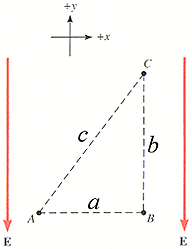# Electric field and change in Voltage question

#### StudentofPhysicsDistances:
a =6.2 cm
b =7.7 cm
c =9.9 cm
E=3650 N/C

Question 1: What is VB - VA? (Between points A & B)I found this to equal 0 as the E field moves about the Y axis. This was correct.

Question 2: VC - VB? (between points B & C) I found this to be 281.05V using Delta V = E x -Delta S. This was also correct.

Question 3: VA - VC (between points C and A). I can not figure this out.

I'm assuming the pathagorian theorem comes into play, however if one of the sides = 0 for E, then the hypotenus would eqaul 3650.

What am I forgetting?

Last edited:

#### Doc Al

Mentor
Hint: Since VB - VA = 0, then VB = VA.

#### StudentofPhysics

Doc Al said:
Hint: Since VB - VA = 0, then VB = VA.

I'm not seeing how that helps, sorry.

#### Doc Al

Mentor
Wherever you see VA, you can replace it with VB. So, VA - VC is equivalent to what? (Then compare with question 2.)

#### StudentofPhysics

Ahhh, I see. Thank you. I'm not entirely sure why that is correct, other than by algebra. Meaning, I get why it works through the transitive property, but if a force moving toward -y acts on a point B that is further away from point A, why the field strength would be equal and opposite.

#### Doc Al

Mentor
StudentofPhysics said:
...but if a force moving toward -y acts on a point B that is further away from point A, why the field strength would be equal and opposite.
First of all, you are finding potential differences, not field strength: The field strength is given as a constant in the -y direction. In calculating potential differences between points, what matters is the displacement in the direction of the field, not merely the distance. After all, the distance between points A and B is 6.2 cm, but the potential difference is zero because the displacement is perpendicular to the field. Similarly, between C and A, and between C and B the potential difference is the same, since only the y-component of the displacement counts.

### The Physics Forums Way

We Value Quality
• Topics based on mainstream science
• Proper English grammar and spelling
We Value Civility
• Positive and compassionate attitudes
• Patience while debating
We Value Productivity
• Disciplined to remain on-topic
• Recognition of own weaknesses
• Solo and co-op problem solving